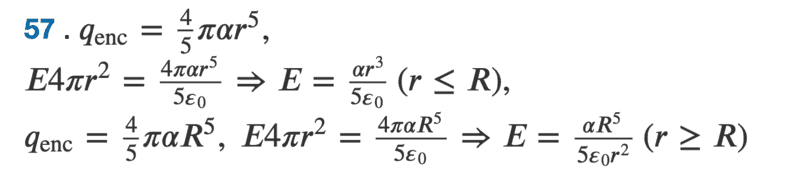# E-field of solid sphere with non-uniform charge density

• mncyapntsi

#### mncyapntsi

Homework Statement
Charge is distributed throughout a spherical volume of radius R with a density
where is a constant. Determine the electric field due to the charge at points both inside and outside the sphere.
Relevant Equations
netEflux = qin/e0, and EA = qin/e0
Hi! I've been trying to attempt this problem over here but the solutions state that the solution is this below?However, from integrating the density and then plugging it into Gauss's law, I get the exact same thing, except a 15 instead of a 5. Could any please help point out if there is an error on my part, or if there is perhaps a type on the solutions?
Thanks a lot!

Your post is missing many relevant equations

And we can't tell where your mistakes, if any, are if you don't show us your work.

You got to tell us what is the given function of the charge density. I suspect that it is something that is spherically symmetrical. Generally when you write for a homework problem here in PF, it is of most importance to give the exact statement of the problem as it is given to you or written in the book you using.

Edit: Is the given charge density $$\rho=ar^2$$? If yes then the book solution is correct.

BUT as others have already said, you must show us your own solution if you want us to pinpoint your mistakes

Last edited: